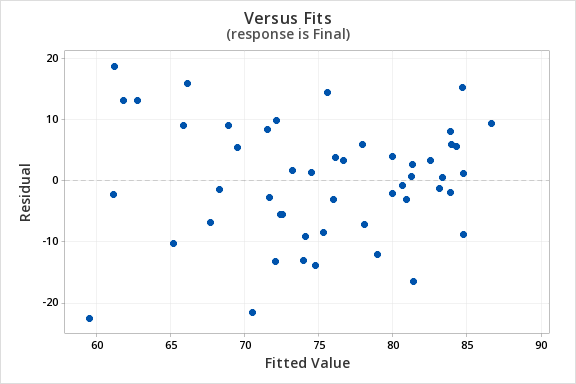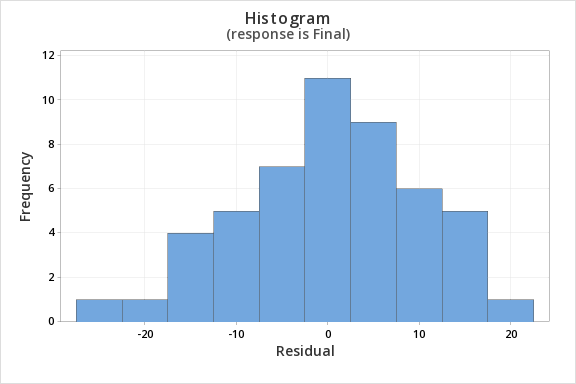# 12.3.4.1 - Example: Quiz and exam scores

Construct a model using quiz averages to predict final exam scores.

This example uses the exam data set found in this Minitab file: Exam.mpx

1. Check assumptions and write hypotheses
• $$H_0\colon \beta_1 =0$$
• $$H_a\colon \beta_1 \neq 0$$

The scatterplot below shows that the relationship between quiz average and final exam score is linear (or at least it's not non-linear).The plot of residuals versus fits below can be used to check the assumptions of independent errors and equal error variances. There is not a significant correlation between the residuals and fits, therefore the assumption of independent errors has been met. The variance of the residuals is relatively consistent for all fitted values, therefore the assumption of equal error variances has been met.Finally, we must check for the normality of errors. We can use the normal probability plot below to check that our data points fall near the line. Or, we can use the histogram of residuals below to check that the errors are approximately normally distributed.Now that we have check all of the assumptions of simple linear regression, we can examine the regression model.

2. Calculate the test statistic
We will use the coefficients table from the Minitab output.
##### Coefficients
Term Coef SE Coef T-Value P-Value VIF
Constant 12.1 11.9 1.01 0.315
Quiz_Average 0.751 0.141 5.31 0.000 1.00
$$t = 5.31$$
3. Determine the p-value

$$p=0.000$$

4. Make a decision

$$p < \alpha$$, reject the null hypothesis

5. State a "real world" conclusion

There is evidence that that students' quiz averages can be used to predict their final exam scores in the population.